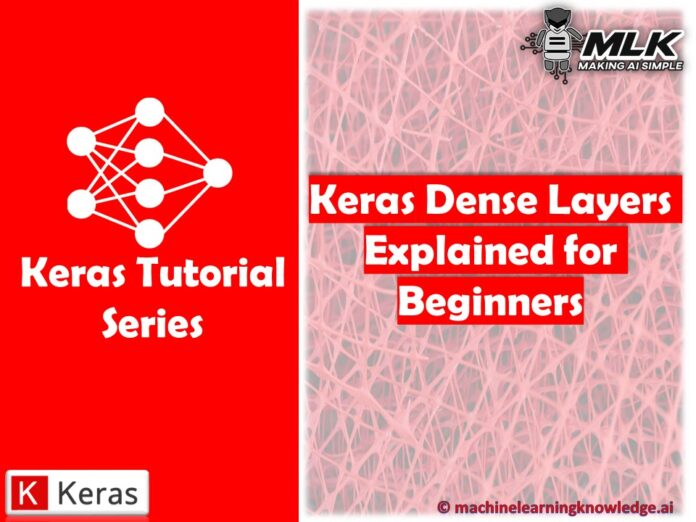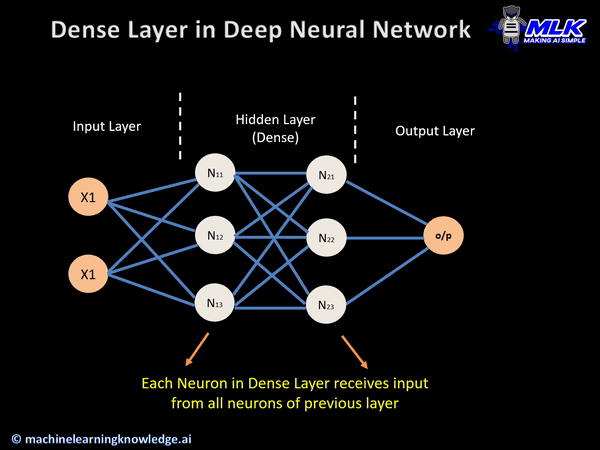# Keras Dense Layer Explained for Beginners## Introduction

In this Keras tutorial, we are going to learn about the Keras dense layer which is one of the widely used layers used in neural networks. We will give you a detailed explanation of its syntax and show you examples for your better understanding of the Keras dense layer.

### What is a Dense Layer in Neural Network?

The dense layer is a neural network layer that is connected deeply, which means each neuron in the dense layer receives input from all neurons of its previous layer. The dense layer is found to be the most commonly used layer in the models.

In the background, the dense layer performs a matrix-vector multiplication. The values used in the matrix are actually parameters that can be trained and updated with the help of backpropagation.

The output generated by the dense layer is an ‘m’ dimensional vector. Thus, dense layer is basically used for changing the dimensions of the vector. Dense layers also applies operations like rotation, scaling, translation on the vector.

### Syntax

keras.layers.Dense(units, activation=None, use_bias=True, kernel_initializer=’glorot_uniform’, bias_initializer=’zeros’, kernel_regularizer=None, bias_regularizer=None, activity_regularizer=None, kernel_constraint=None, bias_constraint=None)

### Keras Dense Layer Parameters

Let us see different parameters of dense layer function of Keras below –

#### 1. Units

The most basic parameter of all the parameters, it uses positive integer as it value and represents the output size of the layer.

It is the unit parameter itself that plays a major role in the size of the weight matrix along with the bias vector.

#### 2. Activation

The activation parameter is helpful in applying the element-wise activation function in a dense layer. By default, Linear Activation is used but we can alter and switch to any one of many options that Keras provides for this.

#### 3. Use_Bias

Another straightforward parameter, use_bias helps in deciding whether we should include a bias vector for calculation purposes or not. By default, use_bias is set to true.

#### 4. Initializers

As its name suggests, the initializer parameter is used for providing input about how values in the layer will be initialized. In case of the Dense Layer, the weight matrix and bias vector has to be initialized.

#### 5. Regularizers

Regularizers contain three parameters that carry out regularization or penalty on the model. Generally, these parameters are not used regularly but they can help in the generalization of the model.

#### 6. Constraints

This last parameter determines the constraints on the values that the weight matrix or bias vector can take.

### Keras Dense Layer Operation

The dense layer function of Keras implements following operation –

output = activation(dot(input, kernel) + bias)

In the above equation, activation is used for performing element-wise activation and the kernel is the weights matrix created by the layer, and bias is a bias vector created by the layer.

Keras dense layer on the output layer performs dot product of input tensor and weight kernel matrix.

A bias vector is added and element-wise activation is performed on output values.

## Keras Dense Layer Examples

We will show you two examples of Keras dense layer, the first example will show you how to build a neural network with a single dense layer and the second example will explain neural network design having multiple dense layers.

### 1. Building Shallow Neural Network with Keras Dense Layer

Now let’s see how a Keras model with a single dense layer is built. Here we are using the in-built Keras Model i.e. Sequential.

First, we provide the input layer to the model and then a dense layer along with ReLU activation is added.

The output layer also contains a dense layer and then we look at the shape of the output of this model.

In :
```import tensorflow as tf

# Create a `Sequential` model and add a Dense layer as the first layer.
model = tf.keras.models.Sequential()

# Now the model will take as input arrays of shape (None, 16)
# and output arrays of shape (None, 32).
# Note that after the first layer, you don't need to specify
# the size of the input anymore:

model.output_shape
```
Output:
`(None, 32)`

### Building Deep Neural Network with Keras Dense LayersIn this example, we look at a model where multiple hidden layers are used in deep neural networks. Here we are using ReLu activation function in the neurons of the hidden dense layer.

Remember one cannot find the weights and summary of the model yet, first the model is provided input data and then we look at the weights present in the model.

At last, the model summary displays the information about the input layers, the shape of output layers, and the total count of parameters.

In :
```from keras.models import Sequential
from keras.layers import Dense

model = Sequential(
[
Dense(5, activation="relu"),
Dense(10, activation="relu"),
Dense(15),
]
)  # No weights to be addded here

# Here we cannot check for weights
# model.weights

# Neither we can look at the summary
# model.summary()

# First we must call the model and evaluate it on test data
x = tf.ones((5, 20))
y = model(x)
print("Number of weights after calling the model:", len(model.weights))
```
Output:
```Number of weights after calling the model: 6
```
In :
```# Looking at the Summary of the Model
model.summary()
```
Output:
```Model: "sequential_2"
_________________________________________________________________
Layer (type)                 Output Shape              Param #
=================================================================
dense_4 (Dense)              (5, 5)                    105
_________________________________________________________________
dense_5 (Dense)              (5, 10)                   60
_________________________________________________________________
dense_6 (Dense)              (5, 15)                   165
=================================================================
Total params: 330
Trainable params: 330
Non-trainable params: 0
_________________________________________________________________
```

## Conclusion

We have reached to the end of this Keras tutorial, here we learned about Keras dense layer. We looked at how dense layer operates and also learned about dense layer function along with its parameters. We also throw some light on the difference between the functioning of the neural network model with a single hidden layer and multiple hidden layers.

Reference Keras Documentation

1.yash
•MLK